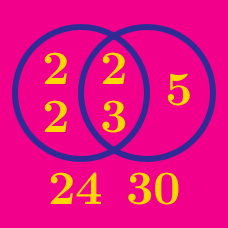Number Theory

# Greatest Common Divisor / Lowest Common Multiple: Level 3 Challenges

If $x$ and $y$ are integers, what is the smallest possible positive value of $30x+18y?$

$\huge \color{#D61F06}{2}, \color{#20A900}{-4}$

Find the lowest common multiple of the two numbers above.

$\LARGE \color{#3D99F6}{0.\bar{3}}, \color{#D61F06}{0.\bar{5}}$

What is the lowest common multiple of the two numbers given above?

Note that $0. \overline{ABC} = 0.ABCABCABC\ldots$.

How many positive integers $a$ are there such that 2027 divided by $a$ leaves a remainder of 7?

Evaluate $\gcd ( 19 ! + 19, 20! + 19 )$.

Details and assumptions

The number $n!$, read as n factorial, is equal to the product of all positive integers less than or equal to $n$. For example, $7! = 7 \times 6 \times 5 \times 4 \times 3 \times 2 \times 1$.

How many ordered pairs of positive integers $(m, n)$ satisfy

$\gcd (m^3, n^2) = 2^2 \cdot 3^2 \text{ and } \text{lcm}(m^2, n^3) = 2^4 \cdot 3^4 \cdot 5^6?$

This problem is shared by Muhammad A.

Details and assumptions

$\gcd(a, b)$ and $\text{lcm}(a, b)$ denote the greatest common divisor and least common multiple of $a$ and $b$, respectively.

×